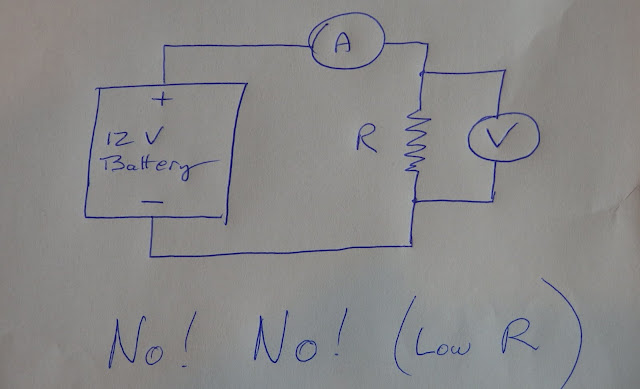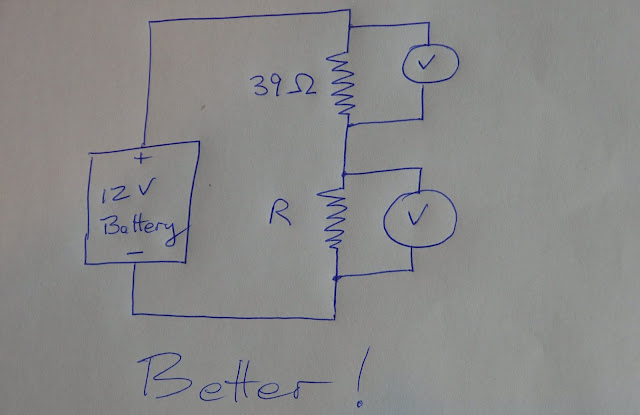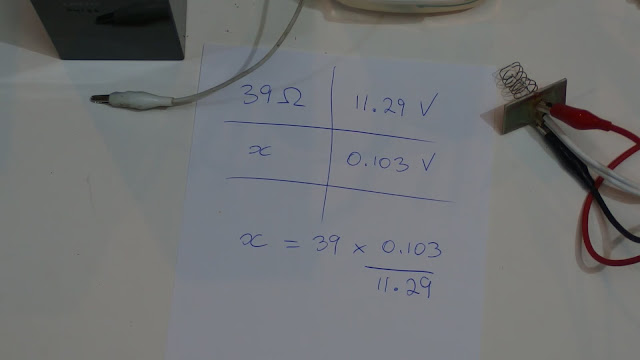## Tuesday, February 9, 2016

### SteamFlash Rocket Motor 04 - What is my (low) resistance?

Getting close to a first test run.
We need to know the resistance of the heater coil.

Simple following of the theory -  R = V divided by I  - gives a circuit like this which will not work for measuring a low resistance. The high current will give heating effects to the point of burnout for either the coil or the battery or even both.Instead I used a standard component resistor that is large enough to get the current down to a moderate level, but still enough current to create a voltage drop across the coil that my meter can accurately measure.  I do not have 2 meters - the one electronic multimeter takes turns measuring the 2 resistances.39 Ohms still lets through enough current (0.3A) to get warm so I run it in a water bath.
Of course you should know that as a general rule we do not mix electricity and water. This is however reasonable with a low voltage battery circuit and clean water.

For future measurements, I plan to use a higher resistance like 390 Ohm. That would give a very low current (0.03A) with no significant heating effect and no need for a water bath. This also gives a very small coil voltage drop like 0.01 V but what I have learned now from doing this is that the modern digital multimeters of today do a good job of measuring very small voltage values.

Photo here shows what the circuit really looks like.
Running here with the multimeter connected to the 39 Ohm resistor.The 39 Ohm resistor has a voltage drop of 11.29 V

The coil has a voltage drop of 0.103 V

The calculation by proportion gives a value of 0.36 Ohm for the coil.
I am surprised at how much higher that is than the 0.23 Ohm I was aiming at - although I was adding  some extra length to "err on the safe side".  But works out well with my aim to start with low power test runs.Question: Why is the coil not running underwater?  Isn't it supposed to be a heating coil?

Answer: In this circuit, the 39 Ohm resistor acts as a power hog, taking nearly all the power so there is very little power and therefore heat dissipation happening with the coil.

The coil will get its turn to get the power in our next episode.